# MATHEMATICS —2004 (Set I— Delhi)

SECTION A

Q. 1. Solve the following system of linear equations: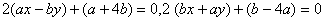Ans.Or

Two years ago, a father was five times as old as his son. Two years later, his age will be 8 more than three times the age of the son. Find the present ages of father and son.

Ans. (12 years, 10 years)

Q.2. Solve forAns.Q. 3. The HCF and LCM of two polynomials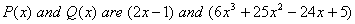respectively. If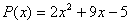, determineAns.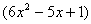Or

If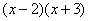is the GCD of the polynomials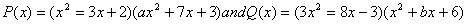Ans. (a=2, b=--5)

Q. 4. If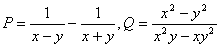and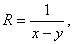express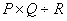as a rational expression in lowest terms.

Ans.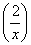Q. 5. The 7 th term of an AP. Is 32 and its 13 th term is 62. Find the A.P.

Ans. (2, 7, 12, 17.)

Q. 6. Find the sum of the first 25 terms of an A.P. whose n th term is given by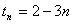Ans. (-925)

Q. 7. A radio is available for Rs. 1,500 cash or Rs. 360 as cash down payment followed by three equal monthly instalments of Rs. 390. Find the rate of interest charged under the instalment scheme.

Ans. (16%

Q. 8. In Fig. 1, D is a point on the side BC of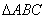such that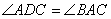Prove thatQ. 9. A loan of Rs. 11,000 has to be repaid in two equal annual instalments. If the interest is charged at the rate of 20% per annum, compounded annually, find the amount of each instalment.

Ans. (Rs.7, 200)

Q. 10. In Fig. 2, PQ is a diameter of a circle with centre 0. If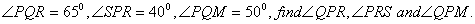Ans. (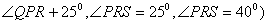SECTION B

Q. 11. Solve the following system of linear equations graphically: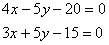Determine the vertices of the triangle formed by the lines, representing the above equations, and the y-axis. Ans. [A(0,3), B(5,0) , C(0,- 4)]

Q. 12. Solve forgiven that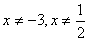Ans. (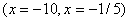Q. 13. A solid metallic sphere of diameter 21 cm is melted and recasted into a number of smaller cones, each of diameter 7 cm and height 3 cm. Find the number of cones so formed.

Ans. (126 cones)

Q. 14. If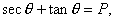prove that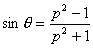Or

Without using trigonometric tables, evaluate the following:Ans. (1)

Q. 15. Construct a triangle ABC in which BC = 6.5 cm,and median AD = 4.5 cm.

Q. 16. Show that the points A(5, 6), B(1, 5), C(2, 1) and D(6, 2) are the vertices of a square.

Q. 17. Determine the ratio in which the point P(m, 6) divides the join of A(-4, 3) and B(2, 8). Also find the value of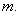Ans.Or

A(3, 2) and B(-2, 1) are two vertices of a triangle ABC, whose centroid G has coordinates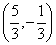. Find the coordinates of the third vertex C of the triangle.

Ans.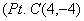Q. 18. The mean of the following frequency distribution is 62.8 and the sum of all frequencies is 50. Compute the missing frequencies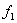and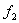:

 Class 0-20 20-40 40-60 60-80 80-100 100-120 Total Frequency 5107 8 50

Ans.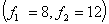Q. 19. A bag contains 5 black, 7 red and 3 white balls. A ball is drawn from the bag at random; Find the probability that the ball drawn is (i) red (ii) black or white (iii) not black

Ans. (7/15, 8/15. 2/3)

Q. 20. The following data shows the expenditure of a person on different items during a month Represent the data by a pie-chart:

 Items of Expenditure Rent Education Food Clothing Others Amount (in Rupees) 2,700 1,800 2,400 1,500 2,400

SECTION C

Q. 21. Prove that the ratio of the areas of two similar triangles is equal to the ratio of the squares of their corresponding sides.

Using the above, do the following:

The areas of two similar triangles ABC and PQR are in the ratio of 9 :16 . If BC = 4.5 cm, find the length of QR.

Q. 22. Prove that the angle subtended by an arc at the centre is double the angle subtended by it at any point on the remaining part of the circle.

Using the above, do the following: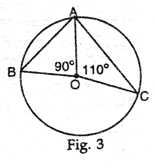In Fig. 3, A, B and C are three points on the circle with centre O such that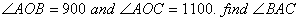Ans.Or

If a line touches a circle and from the point of contact a chord is drawn, prove that the angles which this chord makes with the given line are equal respectively to the angles formed in the corresponding alternate segments. Using the above, do the following: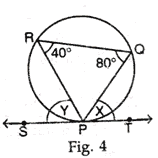In Fig. 4, SPT is a tangent to the circle at P and PQ is a chord of the circle. If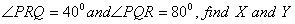Ans.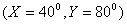Q. 23. If the angle of elevation of a cloud from a point h metres above a lake is a and the angle of depression of its reflection in the lake is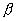, prove that the distance of the cloud from the point of observation is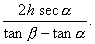Or

From an aeroplane vertically above a straight horizontal plane, the angles of depression of two consecutive kilometre stones on the opposite sides of the aeroplane are found to be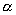and. Show that the height of the aeroplane is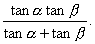Q. 24. A circus tent is cylindrical to a height of 3 m and conical above it. If its base radius is 52.5 m and slant height of the conical portion is 53 m, find the area of the canvas needed to make the tent.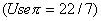Ans. (9735)

Q. 25. Madan Lal has an annual income of Rs.3,30,000 (exclusive of HRA). He contributes Rs.5,000 per month towards his GPF and pays an annual LIC premium of Rs.15,000. He has invested Rs.10,000 in N.S.C's. He pays Rs. 4,500 as income tax per month for the first 11 months. Find his income tax liability for the last month of the financial year.

Use the following for calculating income tax:

 (a) Standard Deduction Gross income Deduction (i) Upto Rs.75,000 (ii) From Rs.75,001 to Rs.5,00,000 (iii) More than Rs,5,00,000 Rs.20,000 40% of gross income Rs.30,000 Rs.20,000 (b) Rates of Taxes Slab Income tax (i) Upto Rs. 50,000 (ii) From Rs. 50,001 to Rs. 60,000 (iii) From Rs. 60,001 to Rs. 1,50,000 (iv) Rs. 1.50,000 and above No tax 10% of the amount exceeding Rs.50,000 Rs.1,000+20% of the amount Exceeding Rs.60,000 Rs.19,000+30% of the amount Exceeding Rs.1,50,000 (c) Rebate in Tax (i) 20% of the savings subject to a maximum of Rs. 14,000 if the gross income is upto Rs. 1,50,000. (ii)15% of the savings subject to a maximum of Rs. 10,500 if the gross income is from Rs. 1,50,001 to Rs. 5,00,000.

Ans.(Rs4,000)

Maths 2004 Question Papers Class X
CBSE 2004 Question Papers Class X